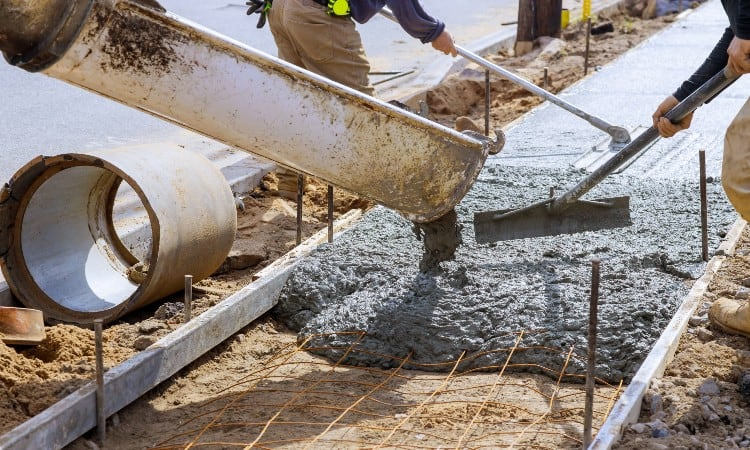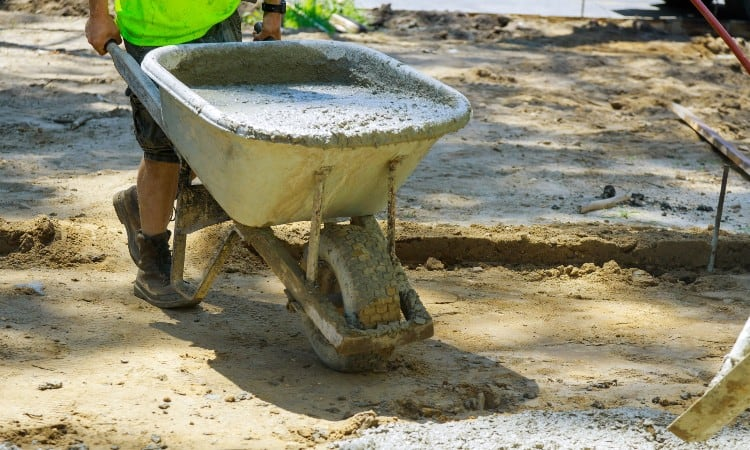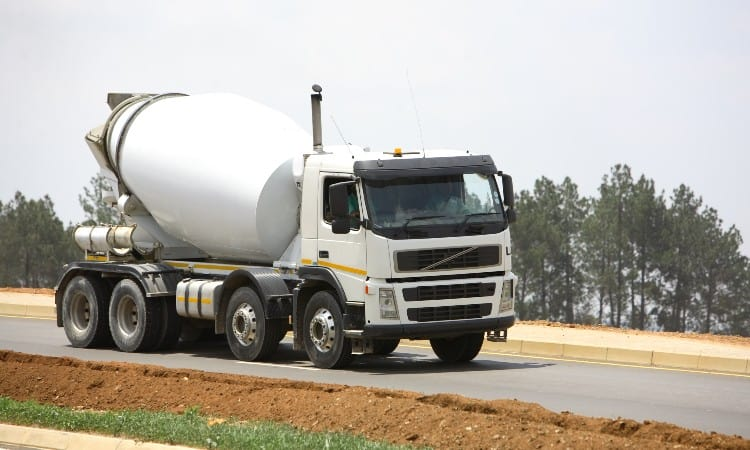# How Much Does a Yard of Concrete Weigh?

As a homeowner, few things are exciting as tackling a renovation or landscaping project you’ve been planning. Concrete plays a role in various builds, including the creation of fantastic patios or foundations for homes and outbuildings. If you need to bring bulk concrete to your property for your project, you may find yourself wondering, “How much does a yard of concrete weigh?”

Typically, a yard of concrete weighs between 3,600 and 4,050 pounds or 1.8 and 2 tons. The weight variance is usually based on whether the concrete is wet or dry. Adding water adds weight, making wet concrete heavier than dry if both are the same volume.

However, whether wet or dry, concrete is a heavy material. Additionally, a yard of concrete is a significant volume, which can create transportation challenges. If you need to get a yard of concrete for your project, here’s what you need to know.## What Is a Yard of Concrete?

When discussing yards in terms of purchasing products like concrete, “yard” refers to a specific volume. A yard – technically a cubic yard – is represented by a cube that’s 1 yard wide, 1 yard high, and 1 yard deep. With 3 feet in a yard, the cube also measures 3 feet wide, 3 feet high, and 3 feet deep, with a total volume of 27 cubic feet.

However, when you purchase a yard of concrete, you aren’t getting a prepackaged cube of the material. Instead, you simply receive the correct total volume. For example, the volume can also be represented by a rectangle 4 feet wide, 1 foot high, and 6.75 feet deep.

## How Much Does a Yard of Concrete Weigh?

The weight of a yard of concrete can vary a bit. However, one usually weighs between 3,600 and 4,050 pounds or 1.8 and 2 tons.

Usually, the lighter end of the range represents dry concrete, such as concrete purchased in bags. The upper end is closer to the weight of correctly saturated concrete, as the water makes the concrete heavier.

## How Much Does 2, 3, 4, 5, 10, 15 Yards of Concrete Weigh?Yards Pounds Tons 2 7,200 to 8,100 3.6 to 4 3 10,800 to 12,150 5.4 to 6 4 14,400 to 16,200 7.2 to 8 5 18,000 to 20,250 9 to 10 10 36,000 to 40,500 18 to 20 15 54,000 to 60,750 27 to 30

## Weight of Concrete Per Cubic Foot

Since a cubic yard of concrete weighs between 3,600 and 4,050 pounds or 1.8 and 2 tons, it’s relatively easy to determine the weight of concrete by cubic foot. There are 27 cubic feet in a yard. By dividing the previous figures by 27, you get the weight of concrete by the cubic foot.

Using that calculation, approximately a cubic foot of concrete weighs between 133 and 150 pounds. If you prefer the weight in tons, a cubic foot of concrete is about 0.067 to 0.074 tons.

## Weight of Concrete Per Cubic Inch

Calculating the weight of concrete per cubic inch is relatively simple. By taking the figures for the weight of concrete per cubic foot and dividing them by 12 (which is the number of inches in a foot), you can get the right amounts.

On the low end, a cubic inch of concrete weighs about 11 pounds. On the upper end, a cubic inch of concrete can weigh around 12.5 pounds.

## How Many 80 LB Bags of Concrete Are in a Yard?

If you’re purchasing concrete in 80 lb. bags, you’re getting dry concrete. As a result, you can use the lower weight in the range, or 3,600 pounds, as an estimate for the number of pounds in a yard as a starting point.

To calculate the number of bags, simply divide 3,600 by 80. When you do, you get 45. As a result, you need 45 80 lb. bags of concrete to get a yard.

## How Many Wheelbarrows in a Cubic Yard of Concrete?The number of wheelbarrow loads in a yard of concrete primarily depends on the size of the wheelbarrow, as that determines its carrying capacity. Generally, small wheelbarrows can carry about 2 cubic feet of material. With a full-size wheelbarrow, the capacity is usually 3 cubic feet.

If you’re using a small wheelbarrow, it will take about 14 wheelbarrow loads to move the concrete. If you have a full-size wheelbarrow, you can move the yard of concrete in about 9 loads.

However, the estimates above are based on filling the wheelbarrow to its maximum capacity on practically every load. As a result, if you don’t haul a full load every time, you’d need to make additional trips to completely move a yard of concrete.

## Is Wet Concrete Heavier Than Dry?

Yes, wet concrete is heavier than dry, the main reason being that water weighs more than air.

When you add water to make the concrete wet, allowing you to use it for your project, you effectively replace the air between the granules with water. As a result, even though the volume remains relatively steady, the weight of the concrete increases. Precisely how much the weight changes depends on the amount of water added.

## How Many Yards of Concrete Are in a Cement Truck?Using a cement truck to transport concrete to the location of your project is beneficial if you require large amounts of concrete. Generally, all cement trucks can safely carry far more than a pickup truck. Plus, a cement truck can move wet concrete, allowing it to arrive ready to pour.

The amount of concrete a cement truck can carry does vary, mainly because cement trucks come in different sizes, each with a different maximum carrying capacity.

The average standard-sized cement truck holds up to 10 yards of concrete. However, larger cement trucks can hold up to 14 yards of concrete. Additionally, mini concrete trucks may be limited to up to 4 yards of concrete.

Now, it’s critical to note that those figures are maximums, not minimums. As a result, if you only need 3 yards of concrete, a cement truck can haul that specific amount.

Additionally, it’s also critical to understand that not all trucking companies will fill a cement truck to its maximum capacity. Since that’s the case, you may need to move up a truck size if the amount you need is right at the limit. For example, if you want 10 yards of concrete, you may need a larger cement truck that can carry at least 11 yards.

## How Much Area Does a Yard of Concrete Cover?Since a yard is a measure of volume, you can figure out how much area it will cover with relative ease. In most cases, when people refer to an area, they’re technically talking about the square footage of space.

How much square footage the concrete covers depends on the thickness of the slab. If you’re pouring depth is 4 inches, the yard of concrete will cover about 81 square feet. If your pouring depth is 6 inches, the concrete will cover around 54 square feet instead.

Doing the calculation is relatively easy. Divide 12 (the number of inches in a foot) by the pouring depth in inches you want to use. Then, multiply that figure by 27 (the number of cubic feet in a yard). The resulting number is the square footage or area that the yard of concrete will cover.

## How to Calculate the Amount of Concrete You Need for Your Project

Determining how much concrete you’ll need for a project is also relatively straightforward. Generally, all you need to do is calculate the total cubic volume of concrete required for your project.

Begin by measuring the length and width in feet of the area you want to cover. Then, multiply those two numbers together to get the square footage.

After that, you need to determine the desired depth for the pour and make sure that figure is in feet. For example, 6 inches is 0.5 feet, while 4 inches is about 0.34 feet. If you aren’t sure what the measurement is in feet, divide the number of inches by 12 (x/12) to get the right figure.

Once you have the depth in feet, multiply it by the square footage. That figure represents the total volume of concrete required. Finally, divide that number by 27 (the total cubic feet in a yard); the resulting figure is the number of yards of concrete your project requires.

Generally, it’s best to round up slightly when you purchase the concrete. Most experts recommend adding at least 10 percent. That ensures you don’t accidentally run short if your calculations were slightly off. It gives you enough concrete to account for variances in the ground, as you’re not dealing with an entirely flat surface. Plus, spillage can happen, so having the extra ensures that it won’t derail your project.

## Concrete Calculator

While you can certainly do the math yourself, you can also take advantage of a concrete calculator to figure out how much concrete you need for your project. The concrete calculator works for slabs and other shapes, like columns, stairs, and more.

Generally, you begin by inputting specific measurements. For a slab, you’ll need to input the desired length, width, and depth. For columns, you’ll put in the diameter and depth.

Once you add the measurements, make sure the desired quantity is correct. Then, you can hit calculate, and you’ll get the results.

Within the results, you’ll usually see the total cubic feet of concrete required for your project and the total cubic yards. It may also include a weight estimate and the total number of bags you’d need to purchase if you buy bags instead of in bulk.

As mentioned above, it’s usually best to round up before making a purchase. Adding approximately 10 percent to the resulting total is typically a safe bet, ensuring you have enough concrete to cover a margin of error.

## Conclusion

Generally, a yard of concrete weighs between 3,600 and 4,050 pounds or 1.8 and 2 tons, with wet concrete weighing more than dry. Additionally, by multiplying the length and width (in feet) of the area you’re covering, multiplying that figure by the desired pouring depth (in feet), and dividing the result by 27, you can estimate the total number of yards required for your project. Then, you’ll have an easier time determining how many bags of concrete you’ll need or how large of a cement truck is required to move your concrete safely.

Did you learn everything you wanted about how much a yard of concrete weighs? If so, please let us know in the comments section below. Also, if you know someone who’s been wondering, “How much does a yard of concrete weigh?” make sure to share the article.